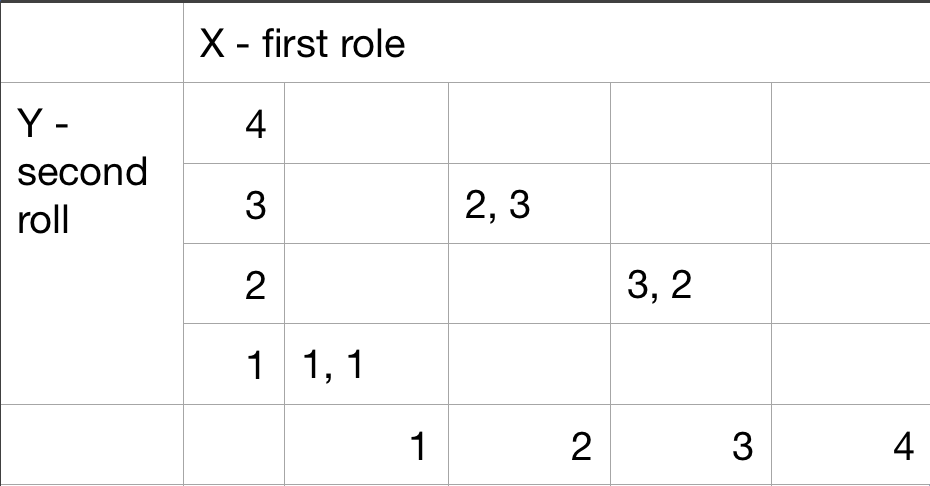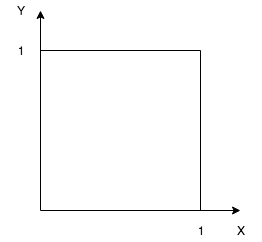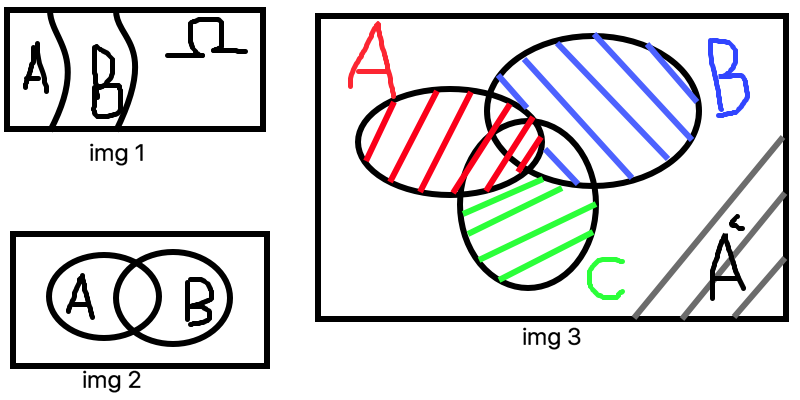# Probability Models and Axioms

## Sample space

• Describe possible outcomes
• Describe beliefs about likelihood outcomes

Possible outcomes:

• List (set) of possible outcomes, $\Omega$ (omega)
• List must be:
• Mutually exclusive
• Collectively exhaustive
• All the “right” granularity

Interpretation and uses of probabilities:

• Measure frequency of the event
• Description of beliefs / betting performance

### Sample space: discrete/finite exampleTable description

Sequential description

Two rolls of a tetrahedral die.

### Sample space: continuous example

$(x, y)$ such that $0 \leqslant x, y \leqslant 1$## Probability axioms

Event
is a subset of the sample space. Probability is assigned to eventsAxioms:

• Nonnegativity: $P(A) \geqslant 0$
• Normalization: $P(\Omega) = 1$
• (Finite) Additivity: if $A \cap B = \varnothing$, then $P(A \cup B) = P(A) + P(B)$

Some simple consequences of the axioms:

• $P(\varnothing) = 0$
• $P(A) + P(A^c) = 1$
• if $A_1, …, A_k$ disjoint, then $P(A \cup … \cup A_k) = \sum_{i=1}^{k}P(A_i)$
• $P(\{S_1, S_2, …, S_k\}) = P(\{S_1\} \cup \{S_2\} \cup … \cup \{S_k\}) = P(\{S_1\}) + … P(\{S_k\}) = P(S_1) + … + P(S_k)$

More consequences of the axioms:

• if $A \subset B$, then $P(A) \leqslant P(B)$ (img 1)
• $P(A \cup B) = P(A) + P(B) - P(A \cap B) = P(A) + P(A^c \cap B)$ (img 2)
• $P(A \cup B) \leqslant P(A) + P(B)$ - union bound
• $P(A \cup B \cup C) = P(A) + P(A^c \cap B) + P(A^c \cap B^c \cap C)$ (img 3)## Probability calculations

### Discrete finite example

Discrete uniform law:

• Assume $\Omega$ finite consist of $n$ equally likely elements
• Assume $A$ consist of $k$ elements, then

$P(A) = k * frac{1}{n}$

every outcome prob == $\frac{1}{16}$

• $P(X = 1) = 4 * \frac{1}{16}$
• let $Z = min(X, Y)$
• $P(Z = 4) = 1/16$
• $P(Z = 2) = 5 * \frac{1}{16}$

### Discrete continuous example

Suppose we have $(x, y)$ such that $0 \leq x, y, \leq 1$

Uniform probability law: Probability = Area

$P(\{(x, y) | x + y \leq 1/2 \})$ = triangle area = $\frac{1}{2} * \frac{1}{2} * \frac{1}{2}$

$P(\{0.5, 0.3 \})$ = area of one point = $0$

### Probability calculations step

• Specify sample space
• Specify a probability law
• Identify an event of interest(if possible - graphical way)
• Calculate …

Let’s we have sample space $\{1, 2, 3, … \}$

$P(n) = \frac{1}{2^n}, n=1, 2..$

$\sum_{n=1}^{\infty} \frac{1}{2^n} = \frac{1}{2} \sum_{n=0}^{\infty} \frac{1}{2^n} = \frac{1}{2} * \frac{1}{1 - 1/2} = 1$

P(outcome is even) = $P(\{2, 4, 6, … \} ) = P(\{2\} \cup \{4\} \cup ..) = P(2) + P(4) + .. =$

$= \frac{1}{2^2} + \frac{1}{2^4} + … = \frac{1}{4}(1 + \frac{1}{4} + \frac{1}{4^2} + …) = \frac{1}{4} * \frac{1}{1 - 1/4} = \frac{1}{3}$

• if $A_1, A_2. A_3$ is an finite sequence of disjoint events, then $P(A_1 \cup A_2 \cup A_3 …) = P(A_1) + P(A_2) + P(A_3)$